# Data Science Posts and Resources

Articles on Data Science

## Neural Networks

Artificial neural networks are mathematical structures that are inspired by the human brain They consist of so-called neurons , which are interconnected with each other. The human brain consists of multiple billions of such neurons. Artificial neural networks use a similar principle.

Laxmi K Soni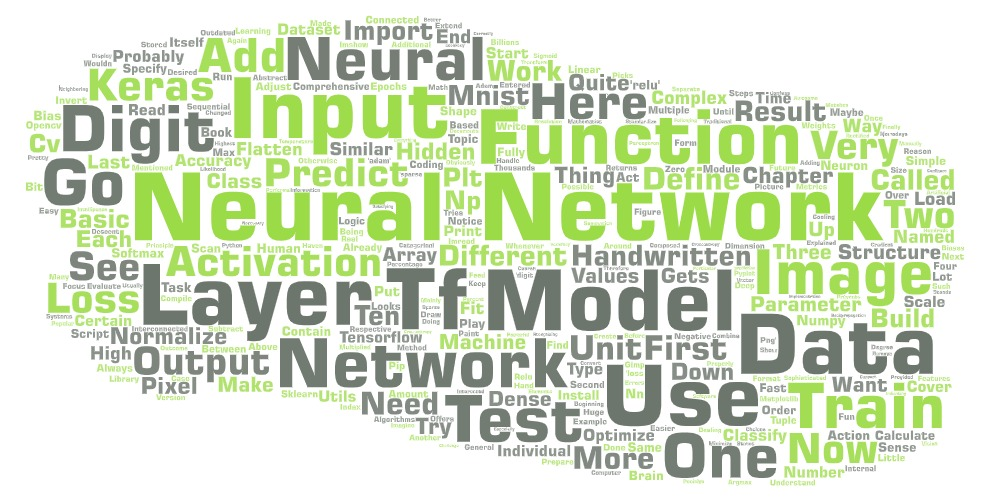## Artificial Neural Networks

Artificial neural networks are mathematical structures that are inspired by the human brain They consist of so-called neurons , which are interconnected with each other. The human brain consists of multiple billions of such neurons. Artificial neural networks use a similar principle.

#### STRUCTURE OF A NEURAL NETWORK

The structure of a neural network is quite simple. In the figure below, we can see multiple layers. The first one is the input layer and the last one is the output layer . In between we have multiple so-called hidden layers . The input layer is for the data that we want to feed into the neural network in order to get a result. Here we put all of the things that are “perceived” by or put into the neural network. For example, if we want to know if a picture shows a cat or a dog, we would put all the values of the individual pixels into the input layer. If we want to know if a person is overweight or not, we would enter parameters like height, weight etc. The output layer then contains the results. There we can see the values generated by the neural network based on our inputs. For example the classification of an animal or a prediction value for something. Everything in between are abstraction layers that we call hidden layers. These increase the complexity and the sophistication of the model and they expand the internal decision making. As a rule of thumb we could say that the more hidden layers and the more neurons we have, the more complex our model is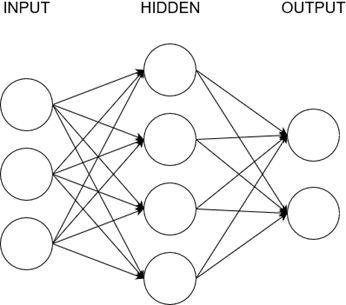In the figure above, we can see three layers. First the input layer , at the end the output layer and in between the hidden layer .

Obviously the input layer is where our inputs go. There we put all the things which are being entered or sensed by the script or the machine. Basically these are our features.

We can use neural networks to classify data or to act on inputs and the output layer is where we get our results. These results might be a class or action steps. Maybe when we input a high temperature into our model, the output will be the action of cooling down the machine.

All the layers between input and output are called hidden layers . They make the model more abstract and more complex. They extend the internal logic.

The more hidden layers and neurons you add, the more sophisticated the model gets.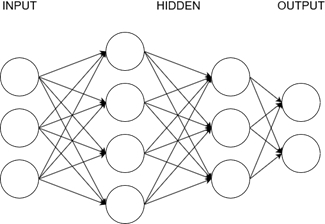Here for example we have two hidden layers, one with four neurons and one with three neurons. Notice that every neuron of a layer is connected to every neuron of the next layer.

#### STRUCTURE OF A NEURON

In order to understand how a neural network works in general, we need to understand how the individual neurons work.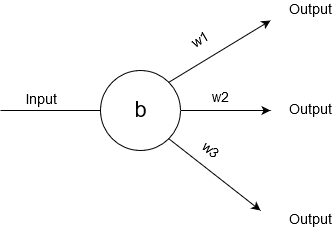As you can see every neuron gets a certain input, which is either the output of a previous neuron or the raw input of the input layer. This input is a numerical value and it then gets multiplied by each individual weight (w1, w2, w3…) . At the end we then subtract the bias (b) .

The result is the output of that particular connection.

These outputs are that forwarded to the next layer of neurons.

The diagram below shows the neural network for computing the square root of a given number.

#### ACTIVATION FUNCTIONS

There are a lot of different so-called activation functions which make everything more complex. These functions determine the output of a neuron. Basically what we do is: We take the input of our neuron and feed the value into an activation function. This function then returns the output value.

#### SIGMOID ACTIVATION FUNCTION

A commonly used and popular activation function is the so-called sigmoid activation function . This function always returns a value between zero and one, no matter what the input is. The smaller the input, the closer the output will be to zero. The greater the input, the closer the output will be to one.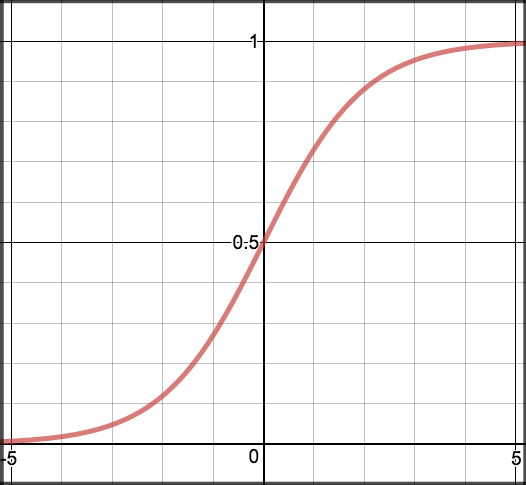The mathematical formula looks like this:

$g(z) = \dfrac{1}{1 + e^{-z}}$

Example in Python:

from keras.models import Sequential
model = Sequential()
..
..


Example in R:

model <- keras_model_sequential()

model %>%
layer_dense(units = 8, activation = 'sigmoid', input_shape = c(4)) %>%
layer_dense(units = 3, activation = 'softmax')

Output layer creates 3 output values, one for each Iris class.
The first layer, which contains 8 hidden notes, on the other hand, has an input_shape of 4.
This is because your training data has 4 columns.


#### RELU ACTIVATION FUNCTION

The probably most commonly used activation function is the so-called ReLU function . This stands for rectified linear unit . This function is very simple but also very useful. Whenever the input value is negative, it will return zero. Whenever it is positive, the output will just be the input.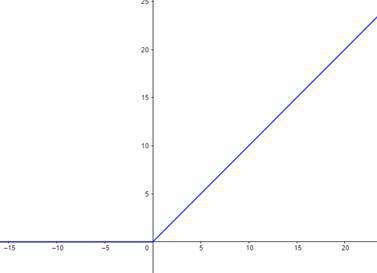$g(z) = \max(0,z)$

Example in Python:

from keras.models import Sequential
(Creates a sequential keras model and add an activation function to it)

model = Sequential()
..
..


Example in R:

model <- keras_model_sequential()

(Output layer creates 3 output values, one for each class.
The first layer, which contains 8 hidden notes, on the other hand, has an input_shape of 4.
This is because your training data has 4 columns.)

model %>%
layer_dense(units = 8, activation = 'relu', input_shape = c(4)) %>%
layer_dense(units = 3, activation = 'softmax')


#### TYPES OF NEURAL NETWORKS

Neural networks are not only different because of the activation functions of their individual layers. There are also different types of layers and networks

##### FEED FORWARD NEURAL NETWORKS

The so-called feed forward neural networks could be seen as the classic neural networks. Up until now we have primarily talked about these. In this type of network the information only flows into one direction – from the input layer to the output layer. There are no circles or cycles.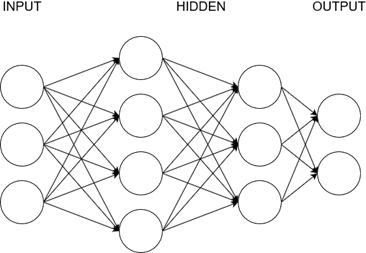##### RECURRENT NEURAL NETWORKS

So-called recurrent neural networks on the other hand work differently. In these networks we have layers with neurons that not only connect to the neurons next layer but also to neurons of the previous or of their own layer. This can also be called feedback .If we take the output of a neuron and use it as an input of the same neuron, we are talking about direct feedback . Connecting the output to neurons of the same layer is called lateral feedback . And if we take the output and feed it into neurons of the previous layer, we are talking about indirect feedback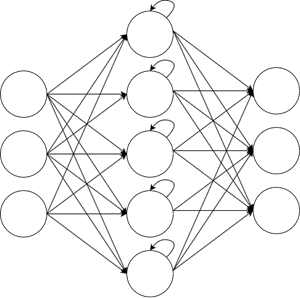The advantage of such a recurrent neural network is that it has a little memory and doesn’t only take the immediate present data into account. We could say that it “looks back” a couple of iterations.

This kind of neural networks is oftentimes used when the tasks requires the processing of sequential data like text or speech. The feedback is very useful in this kind of tasks. However it is not very useful when dealing with image recognition or image processing.

##### CONVOLUTIONAL NEURAL NETWORKS

For this purpose we have the so-called convolutional neural networks . This type is primarily used for processing images and sound. It is especially useful when pattern recognition in noisy data is needed. This data may be image data, sound data or video data. It doesn’t matter.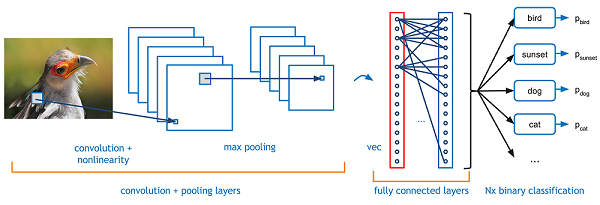Let’s look at a simple example above. Here we have multiple Xs and Os as examples in a 16x16 pixels format. Each pixel is an input neuron and will be processed. At the end our neural network shall classify the image as either an X or an O.

#### SUMMARY

• Activation functions determine the activation of a neuron which then influences the outputs.

• The classic neural networks are feed forward neural networks. The information only flows into one direction.

• In recurrent neural networks we work with feedback and it is possible to take the output of future layers as the input of neurons. This creates something like a memory.

• Convolutional neural networks are primarily used for images, audio data and other data which requires pattern recognition. They split the data into features.

• Usually we use 80% of the data we have as training data and 20% as testing data.

• The error indicates how much percent of the data was classified incorrectly.

• The loss is a numerical value which is calculated with a loss function. This is the value that we want to minimize in order to optimize our model.

• For the minimization of the output we use the gradient descent algorithm. It finds the local minimum of a function.

• Backpropagation is the algorithm which calculates the gradient for the gradient descent algorithm. This is done by starting from the output layer and reverse engineering the desired changes.